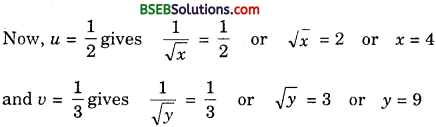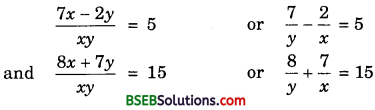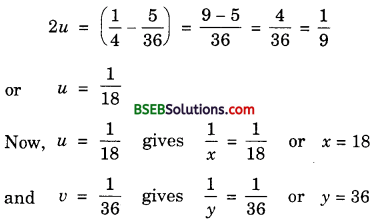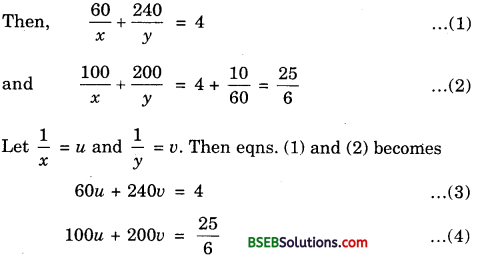# HSSlive: Plus One & Plus Two Notes & Solutions for Kerala State Board

## BSEB Class 10 Maths Chapter 3 Pair of Linear Equations in Two Variables Ex 3.6 Textbook Solutions PDF: Download Bihar Board STD 10th Maths Chapter 3 Pair of Linear Equations in Two Variables Ex 3.6 Book AnswersBSEB Class 10 Maths Chapter 3 Pair of Linear Equations in Two Variables Ex 3.6 Textbook Solutions PDF: Download Bihar Board STD 10th Maths Chapter 3 Pair of Linear Equations in Two Variables Ex 3.6 Book Answers

BSEB Class 10th Maths Chapter 3 Pair of Linear Equations in Two Variables Ex 3.6 Textbooks Solutions and answers for students are now available in pdf format. Bihar Board Class 10th Maths Chapter 3 Pair of Linear Equations in Two Variables Ex 3.6 Book answers and solutions are one of the most important study materials for any student. The Bihar Board Class 10th Maths Chapter 3 Pair of Linear Equations in Two Variables Ex 3.6 books are published by the Bihar Board Publishers. These Bihar Board Class 10th Maths Chapter 3 Pair of Linear Equations in Two Variables Ex 3.6 textbooks are prepared by a group of expert faculty members. Students can download these BSEB STD 10th Maths Chapter 3 Pair of Linear Equations in Two Variables Ex 3.6 book solutions pdf online from this page.

## Bihar Board Class 10th Maths Chapter 3 Pair of Linear Equations in Two Variables Ex 3.6 Books Solutions

 Board BSEB Materials Textbook Solutions/Guide Format DOC/PDF Class 10th Subject Maths Chapter 3 Pair of Linear Equations in Two Variables Ex 3.6 Chapters All Provider Hsslive

## How to download Bihar Board Class 10th Maths Chapter 3 Pair of Linear Equations in Two Variables Ex 3.6 Textbook Solutions Answers PDF Online?

2. Click on the Bihar Board Class 10th Maths Chapter 3 Pair of Linear Equations in Two Variables Ex 3.6 Answers.
3. Look for your Bihar Board STD 10th Maths Chapter 3 Pair of Linear Equations in Two Variables Ex 3.6 Textbooks PDF.
4. Now download or read the Bihar Board Class 10th Maths Chapter 3 Pair of Linear Equations in Two Variables Ex 3.6 Textbook Solutions for PDF Free.

## BSEB Class 10th Maths Chapter 3 Pair of Linear Equations in Two Variables Ex 3.6 Textbooks Solutions with Answer PDF Download

Find below the list of all BSEB Class 10th Maths Chapter 3 Pair of Linear Equations in Two Variables Ex 3.6 Textbook Solutions for PDF’s for you to download and prepare for the upcoming exams:

## BSEB Bihar Board Class 10th Maths Solutions Chapter 3 Pair of Linear Equations in Two Variables Ex 3.6

Question 1.
Solve the following pairs of equations by reducing them to a pair of linear equations:
1. 12𝑥 + 13𝑦 = 2
13𝑥 + 12𝑦 = 136

2. 2𝑥√ + 3𝑦√ = 2
4𝑥√ – 9𝑦√ = – 1

3. 4𝑥 + 3y = 14
3𝑥 – 4y = 23

4. 5𝑥−1 + 1𝑦−2 = 2
6𝑥−1 – 3𝑦−2 = 1

5. 7𝑥−2𝑦𝑥𝑦 = 5
8𝑥+7𝑦𝑥𝑦 = 15

6. 6x + 3y = 6xy
2x + 4y = 5xy

7. 10𝑥+𝑦 + 2𝑥−𝑦 = 4
15𝑥+𝑦 – 5𝑥−𝑦 = – 2

8. 13𝑥+𝑦 + 13𝑥−𝑦 = 34
12(3𝑥+𝑦) – 12(3𝑥−𝑦) = −18
Solution:
1. Taking 1𝑥 = u and 1𝑦 = v, the given system of equations becomes
12u + 13v = 2 or 3u + 2v = 12 ……………… (1)
13u + 12v = 136 or 2u + 3v = 13 ………………. (2)
Multliplying (1) by 3 and (2) by 2, we have:
9u + 6v = 36 ………………. (3)
and 4u + 6v = 26 ………………. (4)
Substracting (4) from (3), we get
5u = 10 or u = 2
Putting u = 2 in (3), we get
18 + 6v = 36 or 6v = 18 or v = 3
Now, u = 2 gives 1𝑥 = 2 or x = 12
and v = 3 gives 1𝑦 = 3 or y = 13
Hence, the solution is x = 12, y = 13.

2. The given system of equation is
2𝑥√ + 3𝑦√ = 2 and 4𝑥√ – 9𝑦√ = – 1
Putting u = 1𝑥√ and v = 1𝑦√, the given equations becomes
2u + 3v = 2 ………………. (1)
and 4u – 9v = – 1 ……………… (2)
6u + 9v = 6 …………………… (3)
Adding (2) and (3), we get
10u = 5 or u = 510 = 12
Putting u = 12 in (1), we get
2 × 12 + 3v = 2 or 3v = 1 or v = 13Hence, the solution is x = 4, y = 9.

3. The given system of equation is
4𝑥 + 3y = 14 …………………. (1)
and 3𝑥 – 4y = 23 ………………. (2)
Multliplying (1) by 4 and (2) by 3, we get
16𝑥 + 12y = 56 ……………….. (3)
and 9𝑥 – 12y = 69 ……………….. (4)
Adding (3) and (4), we get
25𝑥 = 125 or x = 25125 = 15
Putting x = 15 in (1), we get
4 × 5 + 3y = 14 or 3y = 14 – 20
or 3y = – 6 or y = – 2
Hence, the solution is x = 15, y = – 2.

4. Let u = 1𝑥−1 and v = 1𝑦−2. Then, the given system of equations becomes
5u + v = 2 …………………. (1)
and 6u – 3v = 1 ……………….. (2)
Multliplying (1) by 3, we get
15u + 3v = 6 ………………… (3)
Adding (2) and (3), we get
21u = 7 or u = 13
Putting u = 13 in (1), we get
53 + v = 2 or v = 2 – 53 = 6−53 = 13
Now, u = 13 gives
1𝑥−1 = 13 or x – 1 = 3 or x = 4
v = 13 gives
1𝑦−2 = 13 or y – 2 = 3 or y = 5
Hence, the solution is x = 4, y = 5.

5. The given system of equation isLet u = 1𝑥 and v = 1𝑦. Then, the above equations become 7v – 2u = 5 …………… (1)
and 8v + 7u = 15 …………….. (2)
Multliplying (1) by 7 and (2) by 2, we get
49u – 14u = 35 ………………. (3)
and 16v + 14u = 30 ………………. (4)
Adding (3) and (4), we get
65v = 65 or v = 1
Putting v = 1 in (1), we get
7 – 2u = 5 or – 2u = – 2 or u = 1
Now, u = 1 gives 1𝑥 = 1 or x = 1
and v = 1 gives 1𝑦 = 1 or y = 1
Hence, the solution is x = 1, y = 1.

6. The given system of equations is 6x + 3y = 6xy and 2x + 4y = 5xy, where x and y are non-zero.
Since x ≠ 0, y ≠ 0, we have xy ≠ 0.
On dividing each one of the given equatìons by xy, we get
3𝑥 + 6𝑦 = 6 and 4𝑥 + 2𝑦 = 5
Taking 1𝑥 = u and 1𝑦 = v, the above equations become
3u + 6v = 6 …………….. (1)
and 4u + 2v = 5 ………………. (2)
Multiplying (2) by 3, we get
12u + 6v = 15
Subtracting (1) from (3), we get
9u = 15 – 6 = 9 or u = 1
Putting u = 1 in (1), we get
3 × 1 + 6v = 6 or 6v = 6 – 3 = 3
or v = 12
Now, u = 1 gives 1𝑥 = 1 or x = 1
and v = 12 gives 1𝑦 = 12 or y = 2
Hence, the given system of equations has one solution x = 1, y = 2.

7. The given system of equations is
10𝑥+𝑦 + 2𝑥−𝑦 = 4
15𝑥+𝑦 – 5𝑥−𝑦 = – 2
Putting u = 1𝑥+𝑦 and v = 1𝑥−𝑦, the given equations become
10u + 2v = 4 or 5u + v = 2 ………………. (1)
and 15u – 5v = – 2
Putting u = 1𝑥+𝑦 and v = 1𝑥−𝑦, the given equations become
10u + 2v = 4 or 5u + v = 2 ……………… (1)
and 15u – 5v = – 2 ………………… (2)
Multiplying (1) by 5, we get
25u + 5v = 10 ………………… (3)
Adding (2) and (3), we get
40u = 8 or u = 15
Putting u = 15 in (1), we get
5(15) + v = 2 or v = 2 – 1 = 1
Now, u = 15 gives x + y = 5 ……………….. (4)
v = 1 gives 1𝑥−𝑦 = 1
or x – y = 1 …………………. (5)

Question 2.
Formulate the following problems as a pair of equations, and hence find their solutions:
1. Ritu can row downstream 20 km in 2 hours, and upstream 4 km in 2 hours. Find her speed of rowing in still water and the speed of the current.

2. 2 women and 5 men can together finish an embroidery work in 4 days, while 3 women and 6 men can finish it in 3 days. Find the time taken by 1 woman alone to finish the work, and also that taken by 1 man alone.

3. Roohi travels 300 km to her home partly by train and partly by bus. She takes 4 hours, if she travels 60 km by train and the remaining by bus.
If she travels 100 km by train and the remaining by bus, she takes 10 minutes longer. Find the speed of the train and the bus separately.
Solution:
1. Let the speed of the boat in still water be x km/h and the speed of the current be y km/h. Then,
Speed upstream = (x – y) km/h
Speed downstream = (x + y) km/h
Time taken to cover 20 km downstream
= 2 hours
or 20𝑥+𝑦 = 2 or x + y = 10 ……………….. (1)
Time taken to cover 4 km upstream = 2 hours
or 4𝑥−𝑦 = 2 or x – y = 2 ………………. (2)
Adding (1) and (2) we get,
2x = 12 or x = 6
Putting x = 6 in (1), we get
6 + y = 10 or y = 10 – 6 = 4
Hence, the speed of boat in still water = 6km/hr
and speed of current = 4km/hr

2. Let 1 woman can finish the embroidery in x days and 1 man can finish the embroidery in x days and 1 man can finish the embroidery in y days.
Then, 1 woman’s 1 day’s work = 1𝑥
1 man’s 1 day’s work = 1𝑦
∴ 2𝑥 + 5𝑦 = 14 and 3𝑥 + 6𝑦 = 13
Putting 1𝑥 = u and 1𝑦 = v, these equations become
2u + 5v = 14 …………….. (1)
and 3v + 6v = 13 ……………. (2)
Multiplying (1) by 3 and (2) by 2 and substracting, we get
3v = 112 or v = 136
Putting v = 136 in (1), we getThus, 1 woman alone can finish the embroidery in 18 days and 1 man alone can finish it in 36 days.

3. Let the speed of train be x km/hr and speed of bus be y km/hr.Multiplying eqn. (3) by 5 and eqn. (4) by 6, we get∴ Speed of train is 60 km/hr and speed on bus is 80 km/hr.

## Bihar Board Class 10th Maths Chapter 3 Pair of Linear Equations in Two Variables Ex 3.6 Textbooks for Exam Preparations

Bihar Board Class 10th Maths Chapter 3 Pair of Linear Equations in Two Variables Ex 3.6 Textbook Solutions can be of great help in your Bihar Board Class 10th Maths Chapter 3 Pair of Linear Equations in Two Variables Ex 3.6 exam preparation. The BSEB STD 10th Maths Chapter 3 Pair of Linear Equations in Two Variables Ex 3.6 Textbooks study material, used with the English medium textbooks, can help you complete the entire Class 10th Maths Chapter 3 Pair of Linear Equations in Two Variables Ex 3.6 Books State Board syllabus with maximum efficiency.

## FAQs Regarding Bihar Board Class 10th Maths Chapter 3 Pair of Linear Equations in Two Variables Ex 3.6 Textbook Solutions

#### How to get BSEB Class 10th Maths Chapter 3 Pair of Linear Equations in Two Variables Ex 3.6 Textbook Answers??

Students can download the Bihar Board Class 10 Maths Chapter 3 Pair of Linear Equations in Two Variables Ex 3.6 Answers PDF from the links provided above.

#### Can we get a Bihar Board Book PDF for all Classes?

Yes you can get Bihar Board Text Book PDF for all classes using the links provided in the above article.

## Important Terms

Bihar Board Class 10th Maths Chapter 3 Pair of Linear Equations in Two Variables Ex 3.6, BSEB Class 10th Maths Chapter 3 Pair of Linear Equations in Two Variables Ex 3.6 Textbooks, Bihar Board Class 10th Maths Chapter 3 Pair of Linear Equations in Two Variables Ex 3.6, Bihar Board Class 10th Maths Chapter 3 Pair of Linear Equations in Two Variables Ex 3.6 Textbook solutions, BSEB Class 10th Maths Chapter 3 Pair of Linear Equations in Two Variables Ex 3.6 Textbooks Solutions, Bihar Board STD 10th Maths Chapter 3 Pair of Linear Equations in Two Variables Ex 3.6, BSEB STD 10th Maths Chapter 3 Pair of Linear Equations in Two Variables Ex 3.6 Textbooks, Bihar Board STD 10th Maths Chapter 3 Pair of Linear Equations in Two Variables Ex 3.6, Bihar Board STD 10th Maths Chapter 3 Pair of Linear Equations in Two Variables Ex 3.6 Textbook solutions, BSEB STD 10th Maths Chapter 3 Pair of Linear Equations in Two Variables Ex 3.6 Textbooks Solutions,
Share: